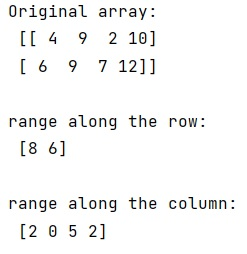# How to find range of a NumPy array elements?

Given a NumPy array, we have to find range of its elements.
Submitted by Pranit Sharma, on March 07, 2023

## Finding range of a NumPy array elements

Suppose that we are given a numpy array of shape 2x4 and we need to calculate the range of the array along the row and the column.

The range of the array along the row can be defined as the max value – min value in that row and similarly for a column.

To find range of a NumPy array elements, we have numpy.ptp() method which stands for peak to peak and it returns the range of values (maximum-minimum) along an axis.

The ptp() preserves the data type of the array. This means the return value for an input of signed integers with n bits (e.g. np.int8, np.int16, etc) is also a signed integer with n bits.

To find the range along the row, we need to set axis = 1 and axis = 0 for the column.

Let us understand with the help of an example,

## Python code to find range of a NumPy array elements

```# Import numpy
import numpy as np

# Creating array
arr = np.array([[4, 9, 2, 10],[6, 9, 7, 12]])

# Display Original array
print("Original array:\n",arr,"\n")

# Finding range along the row
row = np.ptp(arr,axis=1)

# Display result
print("range along the row:\n",row,"\n")

# Finding range along the column
col = np.ptp(arr,axis=0)

# Display result
print("range along the column:\n",col,"\n")
```

Output:Languages: » C » C++ » C++ STL » Java » Data Structure » C#.Net » Android » Kotlin » SQL
Web Technologies: » PHP » Python » JavaScript » CSS » Ajax » Node.js » Web programming/HTML
Solved programs: » C » C++ » DS » Java » C#
Aptitude que. & ans.: » C » C++ » Java » DBMS
Interview que. & ans.: » C » Embedded C » Java » SEO » HR
CS Subjects: » CS Basics » O.S. » Networks » DBMS » Embedded Systems » Cloud Computing
» Machine learning » CS Organizations » Linux » DOS
More: » Articles » Puzzles » News/Updates